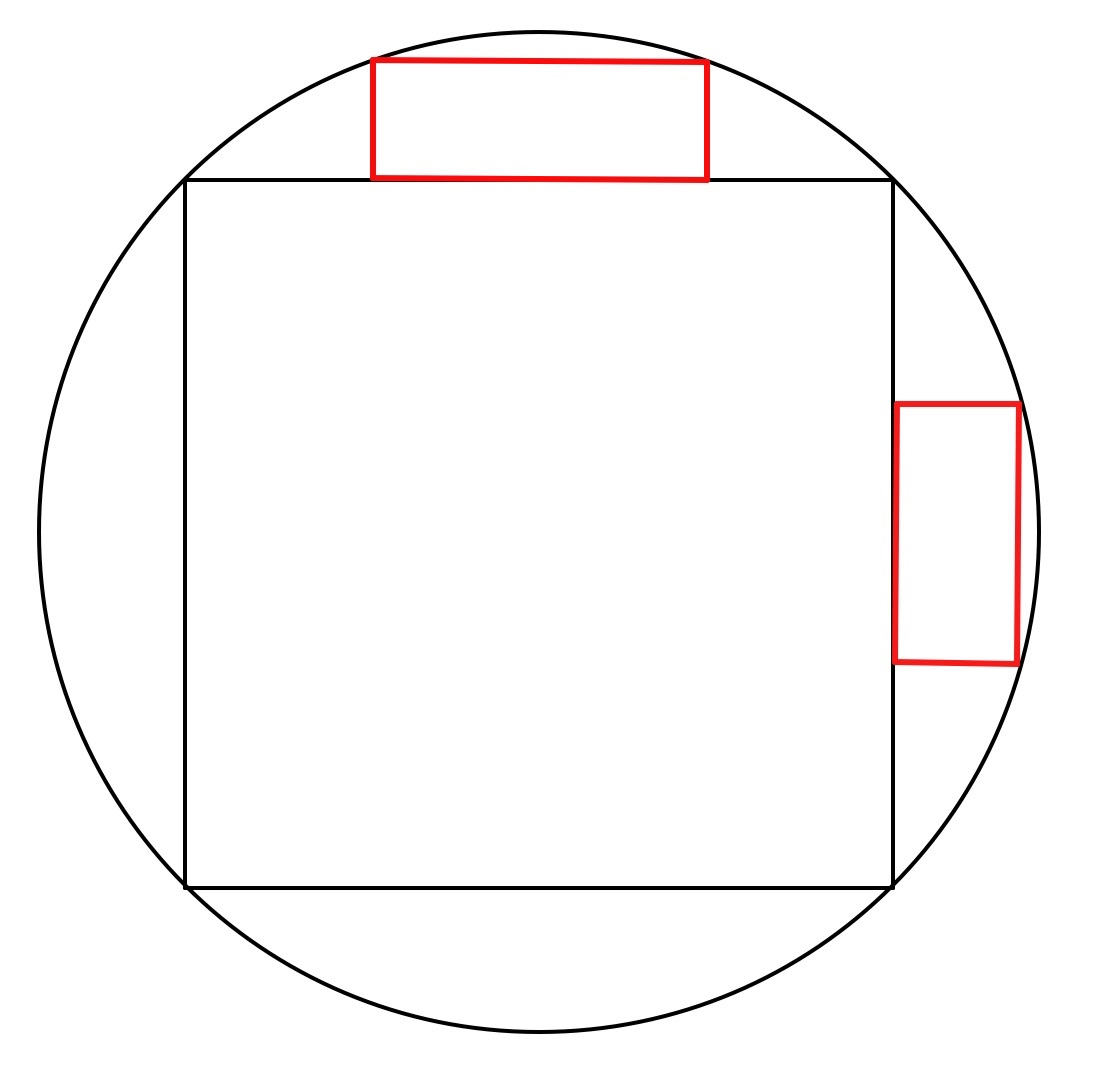# A square and two rectangles in a round hole

Calculus Level 4A square section is cut out of a circle as depicted above. Next, two random rectangles which do not overlap with the other rectangle or the square are cut out of the remaining sectors of the circle. If the maximum possible ratio of the cut out area C to the total area of the circle S is $\dfrac{C}{S}$. Find

$\lceil \left(1000\frac{C}{S} \right) \rceil$

Note: this problem was inspired by one of Sir Brian Charlesworth: Rectangular Pegs in a Round Hole

Assume:

Use of a computer software for ARITHMETIC is allowed.

$\lceil x \rceil$ means the least possible integer greater than or equal to x

Exg: $\lceil 4.1 \rceil=5$

×

Problem Loading...

Note Loading...

Set Loading...